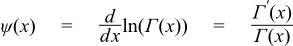#Boost C++ Libraries

...one of the most highly regarded and expertly designed C++ library projects in the world.

This is the documentation for an old version of boost. Click here for the latest Boost documentation.

#### Digamma

##### Synopsis

```#include <boost/math/special_functions/digamma.hpp>
```

```namespace boost{ namespace math{

template <class T>
calculated-result-type digamma(T z);

template <class T, class Policy>
calculated-result-type digamma(T z, const Policy&);

}} // namespaces
```
##### Description

Returns the digamma or psi function of x. Digamma is defined as the logarithmic derivative of the gamma function:The final Policy argument is optional and can be used to control the behaviour of the function: how it handles errors, what level of precision to use etc. Refer to the policy documentation for more details.

There is no fully generic version of this function: all the implementations are tuned to specific accuracy levels, the most precise of which delivers 34-digits of precision.

The return type of this function is computed using the result type calculation rules: the result is of type `double` when T is an integer type, and type T otherwise.

##### Accuracy

The following table shows the peak errors (in units of epsilon) found on various platforms with various floating point types. Unless otherwise specified any floating point type that is narrower than the one shown will have effectively zero error.

Significand Size

Platform and Compiler

Random Positive Values

Values Near The Positive Root

Values Near Zero

Negative Values

53

Win32 Visual C++ 8

Peak=0.98 Mean=0.36

Peak=0.99 Mean=0.5

Peak=0.95 Mean=0.5

Peak=214 Mean=16

64

Linux IA32 / GCC

Peak=1.4 Mean=0.4

Peak=1.3 Mean=0.45

Peak=0.98 Mean=0.35

Peak=180 Mean=13

64

Linux IA64 / GCC

Peak=0.92 Mean=0.4

Peak=1.3 Mean=0.45

Peak=0.98 Mean=0.4

Peak=180 Mean=13

113

HPUX IA64, aCC A.06.06

Peak=0.9 Mean=0.4

Peak=1.1 Mean=0.5

Peak=0.99 Mean=0.4

Peak=64 Mean=6

As shown above, error rates for positive arguments are generally very low. For negative arguments there are an infinite number of irrational roots: relative errors very close to these can be arbitrarily large, although absolute error will remain very low.

##### Testing

There are two sets of tests: spot values are computed using the online calculator at functions.wolfram.com, while random test values are generated using the high-precision reference implementation (a differentiated Lanczos approximation see below).

##### Implementation

The implementation is divided up into the following domains:

For Negative arguments the reflection formula:

```digamma(1-x) = digamma(x) + pi/tan(pi*x);
```

is used to make x positive.

For arguments in the range [0,1] the recurrence relation:

```digamma(x) = digamma(x+1) - 1/x
```

is used to shift the evaluation to [1,2].

For arguments in the range [1,2] a rational approximation devised by JM is used (see below).

For arguments in the range [2,BIG] the recurrence relation:

```digamma(x+1) = digamma(x) + 1/x;
```

is used to shift the evaluation to the range [1,2].

For arguments > BIG the asymptotic expansion:can be used. However, this expansion is divergent after a few terms: exactly how many terms depends on the size of x. Therefore the value of BIG must be chosen so that the series can be truncated at a term that is too small to have any effect on the result when evaluated at BIG. Choosing BIG=10 for up to 80-bit reals, and BIG=20 for 128-bit reals allows the series to truncated after a suitably small number of terms and evaluated as a polynomial in `1/(x*x)`.

The rational approximation devised by JM in the range [1,2] is derived as follows.

First a high precision approximation to digamma was constructed using a 60-term differentiated Lanczos approximation, the form used is:Where P(x) and Q(x) are the polynomials from the rational form of the Lanczos sum, and P'(x) and Q'(x) are their first derivatives. The Lanzos part of this approximation has a theoretical precision of ~100 decimal digits. However, cancellation in the above sum will reduce that to around `99-(1/y)` digits if y is the result. This approximation was used to calculate the positive root of digamma, and was found to agree with the value used by Cody to 25 digits (See Math. Comp. 27, 123-127 (1973) by Cody, Strecok and Thacher) and with the value used by Morris to 35 digits (See TOMS Algorithm 708).

Likewise a few spot tests agreed with values calculated using functions.wolfram.com to >40 digits. That's sufficiently precise to insure that the approximation below is accurate to double precision. Achieving 128-bit long double precision requires that the location of the root is known to ~70 digits, and it's not clear whether the value calculated by this method meets that requirement: the difficulty lies in independently verifying the value obtained.

The rational approximation devised by JM was optimised for absolute error using the form:

```digamma(x) = (x - X0)(Y + R(x - 1));
```

Where X0 is the positive root of digamma, Y is a constant, and R(x - 1) is the rational approximation. Note that since X0 is irrational, we need twice as many digits in X0 as in x in order to avoid cancellation error during the subtraction (this assumes that x is an exact value, if it's not then all bets are off). That means that even when x is the value of the root rounded to the nearest representable value, the result of digamma(x) will not be zero.

 Copyright © 2006 , 2007, 2008 John Maddock, Paul A. Bristow, Hubert Holin, Xiaogang Zhang and Bruno Lalande Distributed under the Boost Software License, Version 1.0. (See accompanying file LICENSE_1_0.txt or copy at http://www.boost.org/LICENSE_1_0.txt)